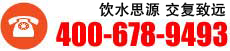# 又快又准!小学数学最常用的速算与巧算技巧1凑十法：

1+9=10

2+8=10

3+7=10

4+6=10

5+5=10

1+2+3+4+5+6+7+8+9+10

1+2=3 3+3=6

6+4=10 10+5=15

15+6=21 21+7=28

28+8=36 36+9=45

45+10=552.凑整法

1+19=20 11+9=20

2+18=20 12+28=40

3+17=20 13+37=50

4+16=20 14+46=60

5+15=20 15+55=70

6+14=20 16+64=80

7+13=20 17+73=90

8+12=20 18+82=100

9+11=20

15+85=100 14+86=100

25+75=100 24+76=100

35+65=100 34+66=100

45+55=100 44+56=100等等3.用已知求未知

1+2+3+4+5+6+7+8+9+10+11+12+13+14+15+16+17+18+19+20

=(1+3+5+7+9+11+13+15+17+19)+(2+4+6+8+10+12+14+16+18+20)

=100+110(这步利用了例2和例3的结果)

=210

5+6+7+8+9+10

=(1+2+3+4+5+6+7+8+9+10)-(1+2+3+4)

(熟练后，此步骤可省略)

=55-10=45

4改变运算顺序

10-9+8-7+6-5+4-3+2-1

=(10-9)+(8-7)+(6-5)+(4-3)+(2-1)

=1+1+1+1+1=5

5带着“+”、“-”号搬家例题：

1-2+3-4+5-6+7-8+9-10+11

=1+3-2+5-4+7-6+9-8+11-10

=1+(3-2)+(5-4)+(7-6)+(9-8)+(11-10)[先减后加]

=1+1+1+1+1+1

=6

## 五年级阅读与写作

五年级学生对阅读没有兴趣，对写作心生...

## 硬笔书法培训

学书法不仅是掌握一种技能，而且是培养...

## 小学词汇与语法

思源教育的小学词汇与语法采用小班教学...

## 四年级阅读与写作

四年级学生对阅读没有兴趣，对写作心生...

## 三年级阅读和写作

思源教育的三年级阅读与写作是根据语文...

## 一年级看图写话

思源教育的一年级看图写话，通过孩子对...

## 少年书法培训

学书法不仅是掌握一种技能，而且是培养...

## 更多校区查询

• 二十年教育经验
• 十余项教育大奖
• 六万余名学员顺利毕业

### 总课程咨询热线：400-678-9493 服务时间：9:00-20:00，双休日照常